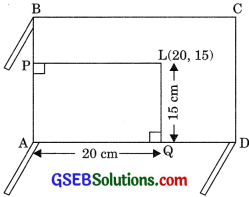# GSEB Solutions Class 9 Maths Chapter 3 Coordinate Geometry Ex 3.1

Gujarat Board GSEB Solutions Class 9 Maths Chapter 3 Coordinate Geometry Ex 3.1 Textbook Questions and Answers.

## Gujarat Board Textbook Solutions Class 9 Maths Chapter 3 Coordinate Geometry Ex 3.1

Question 1.
How will you describe the position of a table lamp on your study table to another person?
Solution:
Consider ABCD is the surface of table. Choose two adjacent edges AD and DC, i.e., AD as x-axis and DC as y-axis. Let lamp pot be placed at point L whose perpendicular distance from AB i.e., y axis is PL = AQ = 20 cm. Hence abscissa is equal to 20 and the perpendicular distance of L from AD is QL = AP 15 cm, therefore ordinate is equal to 15. Hence the coordinates of point L are (20, 15).Question 2.
(Street Plan): A city has two main roads which cross each other at the center of the city. These two roads are along the North-South direction and East-West direction. All the other streets of the city-run parallel to these roads and are 200 m apart. There are 5 streets in each direction. Using 1 cm = 200 m, draw a model of the city on your notebook. Represent the roads! streets by single lines.There are many cross-streets in your model. A particular cross-street is made by two streets, one running in North-South direction and another in the East-West direction. Each cross street is referred to in the following manner: If the second street running in the North-South direction and 5th in the East-West direction meet at some crossing, then we will call this cross-street (2, 5). Using this convention, find:
(i) how many cross-streets can be referred to as (4, 3)
(ii) how many cross-streets can be referred to as (3. 4).
Solution:
Both the cross-streets are shown in the figure.We observed that only one cross street which can he referred as (4, 3) and again, only one which can be referred as (3, 4).